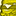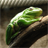# QlikView App Dev

Discussion Board for collaboration related to QlikView App Development.

Announcements
Join this live chat April 6, 10AM EST - QlikView to Qlik Sense REGISTER
cancel
Showing results for
Did you mean:Contributor II

## Set analysis issue with interpreting integer field

Hello,

I have a requirement where I need to compare 2 fields in a set analysis. These 2 fields are integers.

My dimension is just employees. Here is my expression:

``sum({<sk_date ={">=\$([sk_date_start_contract])"}>}[Leave hours])``

sk_date fields are the date in the integer format yyyymmdd.

With this expression the value of sk_date_start_contract seems to be ignore as I got a sum of outbound values.

If I change my expression to  sum({<sk_date ={">=20200601"}>}[Leave hours]) it works as expected. I don't understand what I'm doing wrong.

Afterward I'd like to have 2 boundaries in my expression like so:

sum({<sk_date={">=\$([sk_date_start_contract])<=\$([sk_date_end_contract])}>}[Leave hours]).

Is there a way to acheive this?

Thank you.

Labels (4)

• ### Set Analisys

7 RepliesSpecialist II

Hi HugoR,

Try this:

sum({<sk_date ={"=(sk_date>=[sk_date_start_contract])"}>}[Leave hours])

and when you add in the end date use this:

sum({<sk_date ={"=(sk_date>=[sk_date_start_contract] And sk_date<=[sk_date_end_contract])"}>}[Leave hours])

Hope this helps!Contributor II
Author

Hi Jerem1234,

The idea was good but unfortunately this doesn't works. All my sums end up being 0.

But your expression works with a fixed value like so:

sum({<sk_date ={"=(sk_date>=20200601)"}>}[Leave hours])

This is very frustrating.

Somehow, it seems that the field sk_date_start_contract can't be read.MVP

Two things are coming in my mind.

• Your date field formatting. How have you created it in the script? Probably using date(....., 'YYYYMMDD'). If so, they are not really 20200601 at the backend as number
• Do you want to compare these date fields line-by-line or against a certain date value?

If you could share a sample explaining the expected output - it could probably be a faster resolution for you.Contributor II
Author

Hi @tresesco ,

• My date fields come directly from the database. That's how they are registered in our system.
• My sk_date needs to be compared to the sk_date_start_contract which is a fixed field for each employee

Here is a bit more explanation:

My first table is the contract_table with the following fields:

• id_employee
• sk_date_start_contract
• sk_date_end_contract

This table contain only 1 line by employee.

The second table is the work_time_table:

• id_employee
• sk_date
• work_time
• leave_time
• sick_time

This table as 1 line per day for every employee. Note that they can have some data for dates out of their contract because they may have switch contract at some point. The contract_table has only the contract that is needed for this requirement so there is no duplicate id_employee.

I need to calculate the total leave_time between the start and the end of the contract. that's why I'm filtering the sk_date field on the sk_date_start_contract field.

I hope this help understand the problem better.MVP

Are you using a chart (may be dimension being Employee) for this calculation report? If so, you should try with IF in expression rather than set analysis.Contributor II
Author

Yes I'm using a chart and the dimension is the id_employee.

How would you handle it with a if?

I tryied the following:

=sum(if (sk_date >= sk_date_start_contract and sk_date <= sk_date_end_contract,leave_time)

But it deduplicate the leave_time. For each sk_date it sum all the leave_time inbound.MVP

May be try with distinct qualifier in sum, like : Sum(Distinct( If....

Or, may be using avg() for leave time. However,  that would require an aggr().